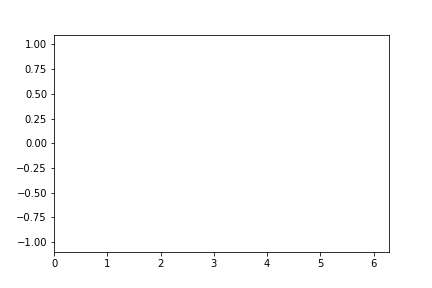# 零基础学习 matplotlib （五）| 绘制酷炫的gif动态图

## 1. 准备工作

magick --version


## 2. 绘制原理

matplotlib 给我们提供了一个函数，animation.FuncAnimation 用它我们就可以很轻松的完成这些画面展示。最后才能交由 ImageMagick 来处理。

# 导入相关模块
import numpy as np
import matplotlib.pyplot as plt
from IPython.display import Image
from matplotlib.animation import FuncAnimation

# 生成数据（用于传入updata函数）
def data_gen():
pass

# 初始化图像（譬如 坐标范围）
def init():
pass

# 将最新数据添加到图像中
def update(data):
pass

# 核心方法入口
ani = FuncAnimation()

# 保存 gif 动态图
ani.save('ming.gif',writer=writer)

# 将 gif 图展示在页面上
Image(url='./ming.gif')


## 3. 方法参数

• fig：进行动画绘制的figure
• init_func：自定义开始帧，即传入刚定义的函数init
• interval：更新频率，以ms计。
• blit：选择更新所有点，还是仅更新产生变化的点。应选择True。
• func：接收来自 frames 函数传来的 frame 值，作为更新图像最新数据。
• frames：可接收对象有 iterable, int, generator function, or None。用途生成数据传递给func函数

## 4. 实战讲解

import matplotlib.pyplot as plt
plt.rcParams['animation.convert_path'] = \
'E:\Program Files\ImageMagick-7.0.8-Q16\magick.exe'

from matplotlib.animation import ImageMagickFileWriter
writer = ImageMagickFileWriter()


# 导入相关模块
import numpy as np
import matplotlib.pyplot as plt
from IPython.display import Image
from matplotlib.animation import FuncAnimation

fig, ax = plt.subplots()
xdata, ydata = [], []
# 注意这边的 "," 不能省
ln, = ax.plot([], [], 'r-', animated=False)

# 初始化图像（譬如 坐标范围）
def init():
ax.set_xlim(0, 2*np.pi)
ax.set_ylim(-1.1, 1.1)
# 注意这边的 "," 也不能省
return ln,

# 将最新数据添加到图像中
def update(frame):
xdata.append(frame)
ydata.append(np.sin(frame))
ln.set_data(xdata, ydata)
# 注意这边的 "," 也不能省
return ln,

# 核心方法入口
ani = FuncAnimation(fig,
update,
frames=np.linspace(0, 2*np.pi, 50),
interval=5,
init_func=init,
blit=True)

# 保存 gif 动态图
ani.save('ming.gif',writer=writer)

# 将 gif 图展示在页面上
Image(url='./ming.gif')